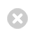Close

MPHY0021: Research Software Engineering With Python

HomeWe've seen already during the course that some operators behave differently depending on the data type.

For example, + adds numbers but concatenates strings or lists:

In :
4 + 2

Out:
6
In :
'4' + '2'

Out:
'42'

* is used for multiplication, or repeated addition:

In :
6 * 7

Out:
42
In :
'me' * 3

Out:
'mememe'

/ is division for numbers, and wouldn't have a real meaning on strings. However, it's used to separate files and directories on your file system. Therefore, this has been overloaded in the pathlib module:

In :
import os
from pathlib import Path

performance = Path('..') / 'ch08performance'
os.listdir(performance)

Out:
['015mandels.ipynb',
'020numpy.ipynb',
'050scaling.ipynb',
'array_memory.svg',
'list_memory.svg',
'index.md',
'040cython.ipynb',
'010intro.ipynb',
'deque_memory.svg']

The above works because one of the elements is a Path object. Note, that the / works similarly to os.path.join(), so whether you are using Unix file systems or Windows, pathlib will know what path separator to use.

In :
performance = os.path.join('..', 'ch08performance')


Now that we have seen that in Python operators do different things, how can we use + or other operators on our own classes to achieve similar behaviour?

Let's go back to our Maze example, and simplify our room object so it's defined as:

In :
class Room:
def __init__(self, name, area):
self.name = name
self.area = area


We can now create a room as:

In :
small = Room('small', 9)
print(small)

<__main__.Room object at 0x7fa1dcfe8df0>


However, when we print it we don't get much infomation on the object. So, the first operator we are overloading is its string represenation defining __str__:

In :
class Room:
def __init__(self, name, area):
self.name = name
self.area = area
def __str__(self):
return f"<Room: {self.name} {self.area}m²>"

In :
small = Room('small', 9)
print(small)

<Room: small 9m²>


How can we add two rooms together? What does it mean? Let's define that the addition (+) of two rooms makes up one with the combined size. We produce this behaviour by defining the __add__ method.

In :
class Room:
def __init__(self, name, area):
self.name = name
self.area = area
return Room(f"{self.name}_{other.name}", self.area + other.area)
def __str__(self):
return f"<Room: {self.name} {self.area}m²>"

In :
small = Room('small', 9)
big = Room('big', 21)
print(small, big, small + big)

<Room: small 9m²> <Room: big 21m²> <Room: small_big 30m²>


Would the order of how the rooms are added affect the final room? As they are added now, the name is determined by the order, but do we want that? Or would we prefer to have:

small + big == big + small


That bring us to another operator, equal to: ==. The method needed to produce such comparison is __eq__.

In :
class Room:
def __init__(self, name, area):
self.name = name
self.area = area
return Room(f"{self.name}_{other.name}", self.area + other.area)
def __eq__(self, other):
return self.area == other.area and set(self.name.split('_')) == set(other.name.split('_'))


So, in this way two rooms of the same area are "equal" if their names are composed by the same.

In :
small = Room('small', 9)
big = Room('big', 21)
large = Room('superbig', 30)
print(small + big == big + small)
print(small + big == large)

True
False


You can add the other comparisons to know which room is bigger or smaller with the following functions:

Operator Function
< __lt__(self, other)
<= __le__(self, other)
> __gt__(self, other)
>= __ge__(self, other)

Let's add people to the rooms and check whether they are in one room or not.

In :
class Room:
def __init__(self, name, area):
self.name = name
self.area = area
self.occupants = []
self.occupants.append(name)

circus = Room('Circus', 3)


How do we know if John is in the room? We can check the occupants list:

In :
'John' in circus.occupants

Out:
False

In :
class Room:
def __init__(self, name, area):
self.name = name
self.area = area
self.occupants = []
self.occupants.append(name)
def __contains__(self, value):
return value in self.occupants

circus = Room('Circus', 3)

'Terry' in circus

Out:
True

We can add lots more operators to classes. For example, __getitem__ to let you index or access part of your object like a sequence or dictionary, e.g., newObject or newObject["data"], or __len__ to return a number of elements in your object. Probably the most exciting one is __call__, which overrides the () operator; this allows us to define classes that behave like functions! We call these callables.

In :
class Greeter(object):
def __init__(self, greeting):
self.greeting = greeting

def __call__(self, name):
print(self.greeting, name)

greeter_instance = Greeter("Hello")

greeter_instance("Eric")

Hello Eric


We've now come full circle in the blurring of the distinction between functions and objects! The full power of functional programming is really remarkable.

If you want to know more about the topics in this lecture, using a different language syntax, I recommend you watch the Abelson and Sussman "Structure and Interpretation of Computer Programs" lectures. These are the Computer Science equivalent of the Feynman Lectures!

Next notebook shows a detailed example of how to apply operator overloading to create your own symbolic algebra system.